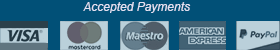﻿ Cubiks Logiks General Intermediate - Cubiks Tests at Aptitude-Test.com
Cubiks Test Prep
Test preparation materials specifically designed for:
• Logiks General Intermediate
• Logiks Numerical
• Logiks Verbal
• Logiks Abstract
• Situational Judgement Test

Only \$29
6 month access
Get Access Now

What you get

• 77 Cubiks practice tests
• All Logiks question categories
• Situational Judgement scenarios
• Clearly Explained Solutions
• Test statistics
• Score progression charts
• Training Assistant
• Arithmetic Trainer
• Vocabulary Trainer
• 24/7 access on all devices

# Cubiks Logiks General Intermediate

Cubiks ability tests are called Cubiks Logiks. Cubiks Logiks are available as an intermediate and an advanced test. There is no “beginners” level.
The intermediate test is considered easier but has more question types in the numerical and verbal sub-tests.

We will cover what you need to know about the Cubiks Logiks General test for the intermediate level.

Free Practice Test

## Logiks General Intermediate

The total test consists of 50 questions with a time limit of 12 minutes. The test includes all three sub-tests: Logiks Verbal, Logiks Numerical, and Logiks Abstract.

### Logiks Verbal

The verbal sub-test of the Logiks contains 24 questions with a time limit of 4 minutes to answer all the questions. The questions in this section cover four different kinds of verbal knowledge and reasoning questions:

Word analogy:
Each question contains four words in pairs but one of the words is missing. You must identify the relationship between the first pair in order to find the missing word in the second pair.

Odd one out:
From a list of words, you have to find the word that is different from the rest.

Antonyms and synonyms:
You are given two words and you must determine if they are synonyms, antonyms or neither.

Deductive reasoning:
Deductive reasoning questions will require you to use your problem-solving and reasoning skills to evaluate arguments and draw logical conclusions.

### Logiks Numerical

The numerical sub-test of the Logiks General Intermediate consists of 16 questions with a time limit of 4 minutes.
The questions in this sub-test are all numerical ability questions and include these types:

Number series:
For these test questions, you will be presented with a series of numbers with one number missing. You will then need to determine the missing number.

Arithmetic ability:
Mental arithmetic questions. These will consist of simple calculations or very basic algebra.

Numerical word problems:
Numerical Word Problems, simply called math word problems, are mathematical questions presented verbally.

### Logiks Abstract

The abstract sub-test of the Logiks General Intermediate test has 10 questions with a time limit of 4 minutes.
The abstract category holds questions that resemble those you would find on an IQ test. The questions contain a series of figures and the test-taker is to choose the figure that is missing in the series or the figure that would complete the series.

### Advice and need to know information before taking the Logiks Advanced test:

Calculators are allowed when taking the test.

Time constraints in these tests can cause a roadblock for many test-takers. You are very restricted in time, particularly in the verbal and numerical sections. You have an average of 10 seconds to answer each question in these two sub-tests. Therefore, it is important that you do not spend too much time on one question. Work quickly and methodically.

With repeated practice, you will speed your ability to do the tests within the given time.
The verbal, numerical and abstract skills needed to complete the tests might not be as difficult as one might expect but a tight time limit can cause many mistakes. It is, therefore, necessary to practice basic math and verbal skills before taking the test. It is also advisable to do some practice tests daily for a week or more prior to taking the scheduled test.

We offer full test preparation packages for the Cubiks Logiks ability tests with over 1000 questions covering both the Logiks intermediate test and the Logiks advanced test.

Try our free Logiks General Intermediate practice test, we've included each of the question types explained above.

Free Practice Test

Aptitude-test.com is not affiliated with Cubiks. Cubiks and other trademarks are the property of their respective trademark holders.

Cubiks Test Prep
Test preparation materials specifically designed for:
• Logiks General Intermediate
• Logiks Numerical
• Logiks Verbal
• Logiks Abstract
• Situational Judgement Test

Only \$29
6 month access
Get Access Now

What you get

• 77 Cubiks practice tests
• All Logiks question categories
• Situational Judgement scenarios
• Clearly Explained Solutions
• Test statistics
• Score progression charts
• 56 Logiks General Intermediate Tests
• Training Assistant
• Arithmetic Trainer
• Vocabulary Trainer
• 24/7 access on all devices
Cubiks Test Prep
Test preparation materials specifically designed for:
• Logiks General Intermediate
• Logiks Numerical
• Logiks Verbal
• Logiks Abstract
• Situational Judgement Test

Only \$29
6 month access
Get Access Now

What you get

• 77 Cubiks practice tests
• All Logiks question categories
• Situational Judgement scenarios
• Clearly Explained Solutions
• Test statistics
• Score progression charts